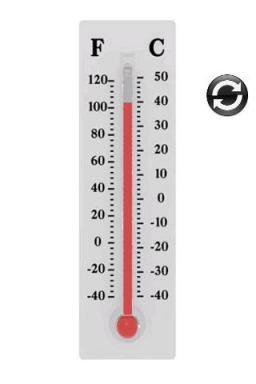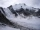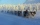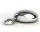# On Sunday

On Sunday the temperature reached a high of 38° above 0. That night it dropped to 23° below 0. What is the difference between the high and low temperatures for Sunday?

Result

d =  61 °C

#### Solution:

$t_{1}=+38 \ ^\circ \text{C} \ \\ t_{2}=-23 \ ^\circ \text{C} \ \\ \ \\ d=t_{1} - t_{2}=38 - (-23)=61=61 ^\circ \text{C}$Our examples were largely sent or created by pupils and students themselves. Therefore, we would be pleased if you could send us any errors you found, spelling mistakes, or rephasing the example. Thank you!

Leave us a comment of this math problem and its solution (i.e. if it is still somewhat unclear...):Be the first to comment!## Next similar math problems:

1. Temperature differenceThe highest temperature of Libya was recorded 58 degree Celsius and the lowest was recorded -88 degree Celsius, what is the temperature difference?
2. LaboratoryMang Elio went to his laboratory at 7:00am And recorded that the temperature was 11.07°C. At lunchtime, the temperature rose by 27.9°C but dropped by 8.05°C at 5:00 PM. What was the temperature at 5:00 PM?
3. The temperature 5The temperature at 4pm was 53° Fahrenheit the temperature dropped 3° each hour from 4pm to 9pm which equation below accurately represents the temperature at 9pm
4. The temperature 2The temperature of Siberia fell13 degrees below 2/3 degrees centigrad. What is the temperature there now?
5. The temperartureThe temperarture is 0 degrees. It drops 5 degrees after 1 hour and then drops 3 degrees more the next hour. What is the temperature after 6 hours?
6. The lowestThe lowest temperature on Thursday was 20°C. The lowest temperature on Saturday was 12°C. What was the difference between the lowest temperatures?
7. 8 degrees8 degrees Celsius it cools down by 9 degrees Celsius. What is the resultant temperature?
8. Melting pointThe melting point of mercury is -36°F and its boiling point is 672°F. What is the difference between the boiling point and the melting point?
9. The temperature 3The temperature is -12 degrees Fahrenheit. During the following 5 hours the temperature decrease 23 degrees Fahrenheit what is the temperature at 11 pm?
10. OpheliaOphelia recorded the temperature of a cold store every two hours. 1. At 6am it was -4°C and at 8am it was -1°C. By how much did the temperature rise? 2. The temperature went up by 5°C in the next two hours. .What was the temperature at 10am?
11. Difference in temperaturesThe temperature in the number line show minus 15 degree celsius and 8 degree celsius. What is the different between the temperatures?
12. Degrees 2The temperature was 3°F and falls four degrees Fahrenheit. What is actually temperature?
13. NoontimeIn the morning temperature was 110°F. By noontime it has gone up by 15°F. What was the noon temperature. (Give your answer in °F)
14. Temperature change 3At 2 p. M. The temperature was 76 degrees Fahrenheit. At 8 p. M. The temperature was 58 degrees Fahrenheit. What was the change in temperature?
15. Temperature increaseIf the temperature at 9:am is 50 degrees. What is the temperature at 5:00pm if the temperature increases 4 degrees Fahrenheit each hour?
16. Temperature 13Work out the temperature after each change. The temperature starts at 6°C and falls by 13°C. The temperature starts at 2°C and falls by 8°C
17. Bitoo and ReenaBitoo ate 3/5 part of an apple and the remaining part was eaten by his sister Reena. How much part of an apple did Renna eat? Who had the larger share? By how much?